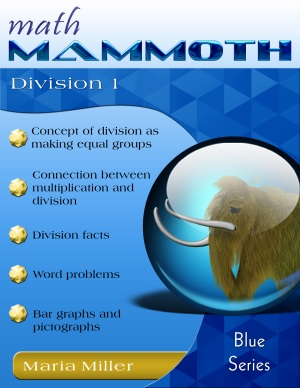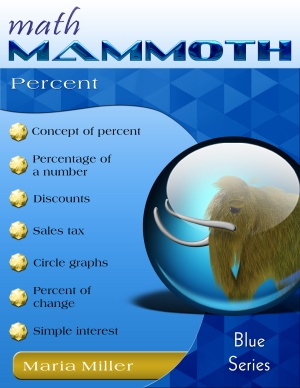Register FREE! Sign In
XP Math Recommends
Math Mammoth Blue Series EBooksMath Mammoth Blue Series ebooks are worktexts on specific math topics for grades 1-7. "Worktext" means that the ebooks contain both explanations of the concepts and lots of varied problems. It is like a textbook and workbook combined.

Each of the Blue Series books concentrates on a few topics only, such as addition & subtraction, place value, clock, measuring, money, multiplication, division, geometry, fractions, decimals, ratios, percent, integers, and so on. This topical arrangement makes the books suitable for many kinds of situations, such as:

• To supplement any other math curriculum;
• As a remedial material for students who are behind;
• As a review material on specific topics.

It is even possible to use the Blue Series books to piece together a complete curriculum; however, I would advice you to consider Light Blue series instead, since it IS designed to be exactly that.The Blue Series books are very affordable as PDF downloads (ebooks): the prices vary from \$2 to \$7.00. They are also available as printed versions.

These books cover all the necessary topics for grades 1-6 and some for grade 7.

The PDF files of these ebooks are enabled for annotating. This means that the student can actually complete the PDF file on the computer, using the typewriter and drawing tools available in Acrobat Reader version 9 or higherm, or on a tablet device such as an iPad, using a PDF app that has annotating tools.

## A List of Blue Series books

The grade-levels mentioned are recommendations only. The books themselves do not mention any grade levels and are usually usable across several grade levels.

Subtraction 1 - grade 1. Addition and subtraction facts within 0-10, difference, "more than", word problems. \$4.25.

Add & Subtract 2 A - grades 1-2. Adding within 0-100 but without carrying or borrowing. Word problems. \$4.50

Add & Subtract 2 B - grade 2. Carrying and borrowing are explained here, with the help of visual models. \$4.25

Add & Subtract 3 - grades 2-3. Adding and subtracting within 0-1000. Lots of mental math techniques included. \$5.00

Add & Subtract 4 - grades 3-4. Adding and subtracting within 0-10,000 and lots of problem solving. \$5.00

### Place value books

Place Value 1 - grade 1. Tens and ones. This book uses a 100-bead abacus, a visual model of base-ten blocks, number lines and a 100-chart. \$3.00

Place Value 2 - grade 2. Three-digit numbers, comparing, rounding. \$2.00

Place Value 3 - grade 3. Four-digit numbers, comparing, rounding. \$3.00

Place Value 4 - grade 4. Numbers till millions, comparing, rounding. \$2.50

Place Value 5 - grades 5-6. Numbers till trillions, rounding, scientific notation, calculator usage. \$2.00

### Clock and money

Clock - grades 1-3. Covers reading the clock, elapsed time, and calendar. \$4.00

Money - grades 1-3. Learn to count U.S. coins, count change, and solve problems. \$3.25

(British Money) - years 2-4. Same as above for UK coins and notes (pounds and pence). \$3.25

(Australian Money) - years or grades 1-3. Same as above but using Australian cents and bills. \$3.25

(European Money) - grades 1-3. Same as above for Euro coins and bills. \$3.00

### Measuring

Measuring 1 - grades 1-3. Teaches all units of customary and metric systems and some conversions. Lots of hands-on exercises with measuring cups, scales, and so on. \$4.50

Measuring 2 - grades 4-5. Teaches all units of customary and metric systems and conversions between the units, including the usage of decimals and fractions with measuring units. \$4.00

Metric Measuring - grades 1-5. This book contains all the lessons from the Measuring 1 and Measuring 2 books (above) that deal with metric measuring units. \$4.50

### Multiplication and division books

Multiplication 1 - grades 2-3. Multiplication concept, word problems, and a system to memorize the multiplication tables. \$5.00

Division 1 - grade 3. Concept of division, basic division facts by one-digit numbers, remainder, word problems. \$3.50

Multiplication 2 - grade 4. Multi-digit multiplication and problem solving. \$4.25

Division 2 - grade 4. Long division, finding fractional parts of a quantity, and problem solving. \$5.00

Multiplication Division 3 - grades 4-5. Multiplication and division with larger numbers, factoring, primes. \$4.50

### Geometry & Statistics

Early Geometry - grades 1-3. Playing with shapes, symmetry, intro to right angle, area, perimeter, and solids. \$2.75

Geometry 1 - grades 4-5. All basic geometry concepts of elementary school geometry are covered here with lots of drawing-based exercises. \$7.00

Geometry 2 - grades 6-7. Geometry for middle school, concentrating on conceptual understanding. \$5.80

Statistics & Probability - grades 5-7. bar graphs, line graphs, histograms, circle graphs, stem-and-leaf plots, mean, median, mode, simple and compound probability. \$5.30

### Fractions and decimals books

Introduction to Fractions - grades 1-3. This book relies almost completely on visual fraction models, and does not go into the most difficult fraction operations such as adding unlike fractions or division. \$4.00

Fractions 1 - grades 4-5. About half of the important fraction topics, explained with the help of visual models. That's how everyone should study fractions! \$4.50

Fractions 2 - grades 5-6. The second half of fraction topics. \$5.00

Decimals 1 - grades 4-5. Builds a solid foundation to understand decimal numbers of 1-2 decimal digits and some of the operations with them. \$4.25

Decimals 2 - grades 5-6. Explaining all of decimal arithmetic, especially all the "trickiness" involved in decimal multiplication and division. \$4.25

Fractions & Decimals 3 - grade 6. All of fraction and decimal arithmetic, with larger denominators in fractions and up to 6 decimal digits in decimals. \$7.00

### Ratios, proportions, percent & algebraic thinking

The Four Operations (with a Touch of Algebra) - grades 5-6. Review of the four operations with whole numbers, order of operations, long division & multiplication, simple equations & expressions, exponents, introduction to functions. \$6.00

Ratios, Proportions & Problem Solving - grades 5-7. Ratios, proportions, scaling geometric figures, floor plans, solving problems using the bar (block) model: problems with fractional parts or ratios. \$4.00

Percent - grades 6-8. Percent, percentage of number, discounts, sales tax, circle graphs, percent of change, percent of comparison. \$3.50

Integers - grades 5-8. Integers, addition, subtraction, multiplication, division, and coordinate grid problems. \$3.75

## Package deals - Blue Series

1. You can get ALL 39 Blue Series books for a package price of \$95 (download) or \$100 (CD). This gives you over 1,800 (unique) lesson and practice pages. (I'm not including the variants of money & measuring books in this count.)

## FAQ

Is the Blue series a complete curriculum?

It is complete in its coverage of topics for grades 1-6, or actually up to pre-algebra.

Please keep in mind that several of the books in the Blue Series cover material for several grade levels in one book. It is NOT organized exactly by grade levels.

Do all the workbooks come with answer keys?

Yes. All of the Blue Series books have answers appended in the worktext.

Can I buy the books as hardcopies (print versions)?

Yes. The Blue series books are available as printed black-and-white softcopies through Lulu.com.

In which order should I study the Blue Series books?